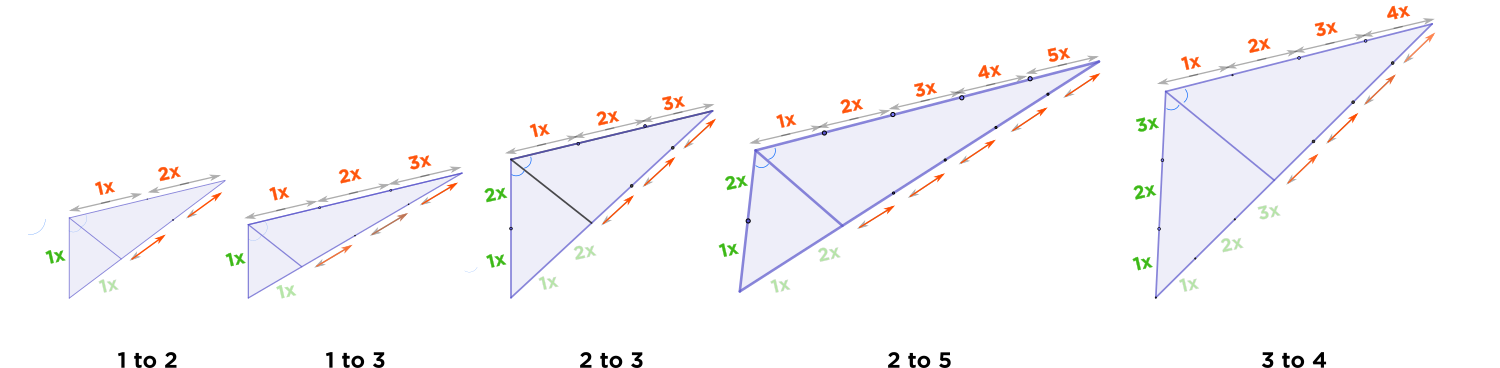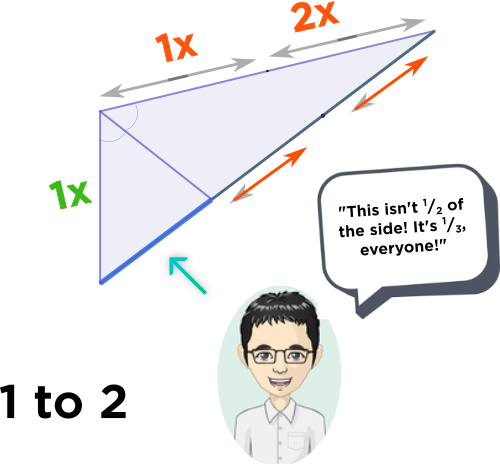# Is it ok if like you provide a simple explanation of the angle bisector theorem

• Module 2 Week 4 Day 13 Challenge Explanation Part 3

Is it ok if like you provide a simple explanation of the angle bisector theorem that say an 8 year old can understand?

• @TSS-Graviser

I've drawn a little picture here of several triangles with angle bisector lines cutting the top-left angle. I hope this helps to visualize what Angle Bisector Theorem means.

For all these triangles, please assume that the line going through the triangle bisects, or cuts the angle it comes out of, perfectly in half.Take a look at the top two sides of the triangle, colored $$\textcolor{green}{\text{green}}$$ and $$\textcolor{red}{\text{red}}.$$

Observe the ratio of the $$\textcolor{green}{\text{green}}$$ and $$\textcolor{red}{\text{red}}$$ sides. I have written this ratio below each triangle, e.g. $$1:2, 1:3,$$ etc.

The Angle Bisector line cuts the bottom side of each triangle into two pieces. What if we want to know exactly how long these pieces are? Well, it's easy! See, if the ratio of the $$\textcolor{green}{\text{green}}$$ and $$\textcolor{red}{\text{red}}$$ sides is $$1:2,$$ then the ratio of the pieces of the cut-up side are in that same ratio, $$1:2.$$

Let me tell you about a very common mistake that some people might make. It's very common to think: "Oh, the $$\textcolor{red}{\text{red}}$$ side is double the $$\textcolor{green}{\text{green}}$$ side, so that means the third side gets cut in half, since that's $$\frac{1}{2}.$$h no, be careful! If the $$\textcolor{red}{\text{red}}$$ side is double the $$\textcolor{green}{\text{green}}$$ side, then the $$\textcolor{green}{\text{green}}$$ side is really $$\frac{1}{3}$$ of the sum of the sides. That means the shorter segment of the cut-up side should be $$\frac{1}{3}$$ of the total side length.I really would have loved to put a proof of the Angle Bisector Theorem here, but it uses some trigonometry and advanced geometry theorems. However, if you'd like to know, I'd be happy to write write it out, because it is surprisingly simple!• @debbie I still don't get how it is not 1/2 when the last picture is appearing a 1/2 ratio?• Hey @victorioussheep! You are correct: on the long side, the ratio of the blue segment to the red segment is indeed 1:2. What the bubble is saying though, is that that blue segment is 1/3 of the WHOLE side, not just the red part.
Hope this makes sense!• @quacker88 Oh...Now it makes sense